Sie sind hier: ICP » R. Hilfer » Publikationen

# 4 Simulation Setup

[681.1.9.1] In this section we discuss how the experiment is represented mathematically. [681.1.9.2] The four mass balance equations (1) are solved numerically. [681.1.9.3] First, equations (6) and (7) are used to eliminate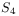and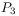. [681.1.9.4] The primary variables areand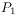. [681.1.9.5] The mass balances are discretized in space by cell centered finite volumes with upwind fluxes. [681.1.9.6] They are discretized in time with a first order implicit fully coupled scheme. [681.1.9.7] The corresponding system of nonlinear equations is solved with the Newton-Raphson method. [681.1.9.8] The whole scheme is implemented in Matlab. [681.1.9.9] The simulation is run with a resolution of one cell per centimeter, i.e. with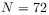collocation points. [681.1.9.10] Details of the algorithm are given elsewhere .

Table 1: List of parameters with units and their numerical values used for simulating the experiment. Note that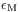is a mathematical regularization parameter, i.e. the limit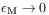is implicit and it has been tested that the numberical results do not change in this limit.
Parameters Units Values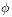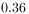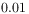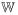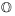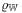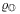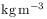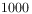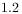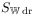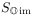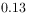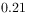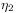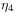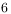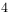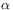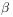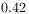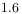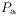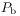Pa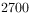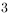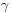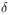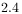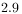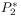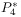Pa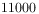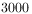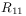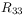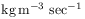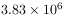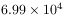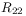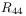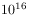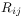,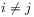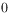[681.1.9.11] Dirichlet boundary conditions for the pressureof the percolating water phase are imposed at the lower boundary (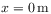), where pressure is determined by the water reservoir. [681.1.9.12] Dirichlet boundary conditions for the atmospheric pressureof the percolating air phase are chosen at the upper boundary (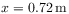) of the column. [681.1.9.13] All the other boundaries are impermeable so that the flux across them must vanish.

[681.1.9.14] The initial conditions are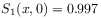,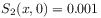,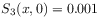,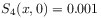for all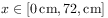[681.1.9.15] Initial conditions for the pressures are not required because of the implicit formulation. [681.1.9.16] Before the protocol for the pressure is started, the system is given one day under hydrostatic water pressure conditions to equilibrate.

[681.1.10.1] The parameters for the simulation are given in Table 1. [681.1.10.2] They were obtained by fitting the primary drainage curve of the capillary pressure saturation relationship obtained in the residual decoupling approximation  to the primary drainage curve of van Genuchten parametrization that  obtained by a fit to data of the first drainage process in the experiment. [681.1.10.3] The van Genuchten parameters in  are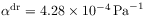,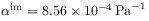,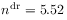,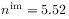,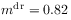,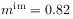,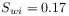,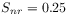. [681.1.10.4] The resulting capillary pressure curves are compared in Figure 2. [681.1.10.5] The viscous resistance coefficients were obtained through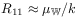,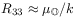, where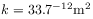was again taken from . [681.1.10.6] The viscous resistance coefficients for the non-percolating phases are assumed to be much larger than those for the percolating phases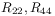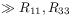. [681.1.10.7] For the time-scale of the experiment the results do not depend on the numerical values of the resistance coefficients given in Table 1 .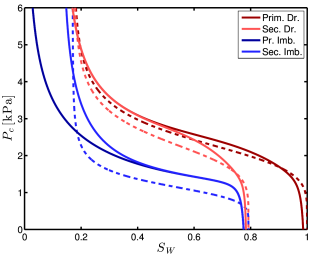Figure 2: Illustration of the capillary pressure-saturation relationship in the residual decoupling approximation of our theory for the parameters given in Table 1 (solid lines) for primary and secondary drainage and imbibition respectively. Also shown are the primary and secondary drainage and the secondary imbibition curve of the hysteresis model used in  (dashed curves).